Latest Banking jobs   »

# Reasoning Ability Quiz For NABARD Grade A 2022- 29th July

Directions (1-15): The question given below is followed by two statements numbered I and II. You have to decide whether the data provided in the statements sufficient to answer the question:

Q1. In the family of five members there are two married couples and three generations, who is the wife of Rajesh?
I. Rajesh is the only child of Sushma and Swaraj. Roshni is the mother of Rahul who is the only grandchild of Swaraj.
II. Rahul is the only grandchild of Sushma. Rajesh is the son of Swaraj, who is the father-in-law of Roshni.

(a) If the data in the statement I alone is sufficient to answer the question, while the data in statement II alone is not sufficient to answer the question.
(b) If the data in the statement II alone is sufficient to answer the question, while the data in statement I alone is not sufficient to answer the question.
(c) If the data either in the statement I alone or in statement II alone is sufficient to answer the question.
(d) If the data in both the statement I and II together are not sufficient to answer the question.
(e) If the data in both the statement I and II together are necessary to answer the question.

Q2. Five different colored bags, viz. Green, Blue, Black, Red and Brown, are stacked one above another, but not necessarily in the same order. Brown bag is stacked at the bottom. Which colored bag is kept at the top of the arrangement?
I. There are two bags kept between Brown and Green. Red bag is not at the top.
II. Only one bag is kept at between the bag keeping at the top and the red bag. The black bag is not kept at the top.

(a) If the data in the statement I alone is sufficient to answer the question, while the data in statement II alone is not sufficient to answer the question.
(b) If the data in the statement II alone is sufficient to answer the question, while the data in statement I alone is not sufficient to answer the question.
(c) If the data either in the statement I alone or in statement II alone is sufficient to answer the question.
(d) If the data in both the statement I and II together are not sufficient to answer the question.
(e) If the data in both the statement I and II together are necessary to answer the question.

Q3. Six people are sitting around a circle facing away from the centre. P is sitting at which position with respect to Q?
I. S sits third to the right of T who sits second to the left of U. S sits second to the right R, who does not sit with P.
II. T and U are immediate neighbours of R, who sits second to the right of Q.

(a) If the data in the statement I alone is sufficient to answer the question, while the data in statement II alone is not sufficient to answer the question.
(b) If the data in the statement II alone is sufficient to answer the question, while the data in statement I alone is not sufficient to answer the question.
(c) If the data either in the statement I alone or in statement II alone is sufficient to answer the question.
(d) If the data in both the statement I and II together are not sufficient to answer the question.
(e) If the data in both the statement I and II together are necessary to answer the question.

Q4. Who is the youngest among six family members (three generations and three married couples) V, W, X, Y, Z and A (Age of each one of them is different)?
I. V is the son-in-law of Y. Z is the husband of A, who is the mother of W. V is not the youngest.
II. A is the eldest. W is the mother of X, who is the granddaughter of A. Y is the husband of Z’s daughter and X’s mother.

(a) If the data in the statement I alone is sufficient to answer the question, while the data in statement II alone is not sufficient to answer the question.
(b) If the data in the statement II alone is sufficient to answer the question, while the data in statement I alone is not sufficient to answer the question.
(c) If the data either in the statement I alone or in statement II alone is sufficient to answer the question.
(d) If the data in both the statement I and II together are not sufficient to answer the question.
(e) If the data in both the statement I and II together are necessary to answer the question.

Q5. Seven persons Kavita, Mahi, Dhurva, Ravi, Shivani, Veena and Lokesh attend the meeting in a week starting from Monday and ends on Sunday. Who attends the seminar on Tuesday?
I. Three persons attend the seminar between Lokesh and Mahi. As many persons attend the seminar before Mahi attends the seminar after Shivani.
II. Ravi attends the seminar before Shivani. Two persons attend the seminar between Ravi and Shivani. Only one person attends the seminar between Veena and Dhurva, who attends the seminar immediately after Mahi.

(a) If the data in the statement I alone is sufficient to answer the question, while the data in statement II alone is not sufficient to answer the question.
(b) If the data in the statement II alone is sufficient to answer the question, while the data in statement I alone is not sufficient to answer the question.
(c) If the data either in the statement I alone or in statement II alone is sufficient to answer the question.
(d) If the data in both the statement I and II together are not sufficient to answer the question.
(e) If the data in both the statement I and II together are necessary to answer the question.

Q6. Six persons M, N, P, H, L and Z are arranged according to their height. Which of the following person is shortest?
I. M is taller than only one person. Z is taller than P and H but shorter than N. H is not the shortest. N is not the tallest.
II. Z is shorter than only one person. H is taller than M and L but shorter than P, who is not the tallest.

(a) If the data in the statement I alone is sufficient to answer the question, while the data in statement II alone is not sufficient to answer the question.
(b) If the data in the statement II alone is sufficient to answer the question, while the data in statement I alone is not sufficient to answer the question.
(c) If the data either in the statement I alone or in statement II alone is sufficient to answer the question.
(d) If the data in both the statement I and II together are not sufficient to answer the question.
(e) If the data in both the statement I and II together are necessary to answer the question.

Q7. Point Q is in which direction with respect to Point L?
I. Point Q is 3m north of Point M. Point R is 4m west of Point Q. Point V is 5m north of Point R. Point X is 6m east of Point V. Point L is 10m south of Point X.
II. Point M is 4m north of Point N. Point R is 3m east of Point N. Point Q is 2m south of Point R. Point V is 5m west of Point M. Point L is 7m north of Point V.

(a) If the data in the statement I alone is sufficient to answer the question, while the data in statement II alone is not sufficient to answer the question.
(b) If the data in the statement II alone is sufficient to answer the question, while the data in statement I alone is not sufficient to answer the question.
(c) If the data either in the statement I alone or in statement II alone is sufficient to answer the question.
(d) If the data in both the statement I and II together are not sufficient to answer the question.
(e) If the data in both the statement I and II together are necessary to answer the question.

Q8. Seven boxes viz. A, B, C, D, E, F and G are kept one above other. How many boxes are kept below box G?
I. Box C is kept third from top. Box D and F are kept together. Box E, which is neither kept adjacent to box F nor box C. Only three boxes are kept between D and C. Box A, which is not kept adjacent to box B, is kept at any place above box G.
II. Only three boxes are kept between box F and A, which is kept second from top. Box B, which is neither kept adjacent to box A nor box F, is kept just below box C. Only three boxes are kept between box D and box C.

(a) If the data in the statement I alone is sufficient to answer the question, while the data in statement II alone is not sufficient to answer the question.
(b) If the data in the statement II alone is sufficient to answer the question, while the data in statement I alone is not sufficient to answer the question.
(c) If the data either in the statement I alone or in statement II alone is sufficient to answer the question.
(d) If the data in both the statement I and II together are not sufficient to answer the question.
(e) If the data in both the statement I and II together are necessary to answer the question.

Q9. Seven persons namely – Praveen, Bablu, Reetu, Shweta, Teenu, Urvi and Vinod have different height. How many persons are taller to Shweta?
I. Shweta is taller to Teenu, Bablu but not as much as Praveen, Reetu. Teenu is taller to Vinod, Urvi but not as much as Reetu, Shweta. Reetu is only short to one person and Urvi is shortest among all.
II. Teenu is taller to Vinod, Urvi but not as much as Reetu, Shweta. Bablu is taller to Teenu, Vinod but not as much as Shweta, Reetu. Praveen is tallest among all. Vinod is only taller to Urvi.

(a) If the data in the statement I alone is sufficient to answer the question, while the data in statement II alone is not sufficient to answer the question.
(b) If the data in the statement II alone is sufficient to answer the question, while the data in statement I alone is not sufficient to answer the question.
(c) If the data either in the statement I alone or in statement II alone is sufficient to answer the question.
(d) If the data in both the statement I and II together are not sufficient to answer the question.
(e) If the data in both the statement I and II together are necessary to answer the question.

Q10. Eight persons namely – A, B, C, D, P, Q, R and S are sitting in a circular table facing centre not necessary in same order. Who sits immediate left of Q?
I. P sits third to the left of A. R, who neither sits adjacent to A nor P, sits second to the left of B. Only one person sits between C and D. A and S sit together.
II. Only one person sits between B and Q. C, who neither sits adjacent to Q nor D, sits facing S. D sits third to the left of B. P sits second to the left of S.

(a) If the data in the statement I alone is sufficient to answer the question, while the data in statement II alone is not sufficient to answer the question.
(b) If the data in the statement II alone is sufficient to answer the question, while the data in statement I alone is not sufficient to answer the question.
(c) If the data either in the statement I alone or in statement II alone is sufficient to answer the question.
(d) If the data in both the statement I and II together are not sufficient to answer the question.
(e) If the data in both the statement I and II together are necessary to answer the question.

Q11. Seven persons T, L, M, D, K, W and H are sitting in a row facing north but not necessarily in the same order. Which of the following persons sit fourth to the left of M?
I. M sits second to the right of T. Two persons sit between T and W. K sits second to the left of W. Only one person sits between H and L. D sits second left of K.
II. Three persons sit between H and K. Either H or K sits at one of the extreme end. T sits left of W. L and T are immediate neighbours. M sits right of K.

(a) If the data in the statement I alone is sufficient to answer the question, while the data in statement II alone is not sufficient to answer the question.
(b) If the data in the statement II alone is sufficient to answer the question, while the data in statement I alone is not sufficient to answer the question.
(c) If the data either in the statement I alone or in statement II alone is sufficient to answer the question.
(d) If the data in both the statement I and II together are not sufficient to answer the question.
(e) If the data in both the statement I and II together are necessary to answer the question.

Q12. Certain number of persons are sitting in a row facing north. Who among the following sits immediate right of C?
I. Only three persons sit between A and D who sits one of the ends. B sits third to the right of C who is not an immediate neighbour of F.
II. C sits fourth to the right of H. E sits second to the left of B who sits one of the extreme ends. F neither immediate neighbour of H nor E.

statement II alone is not sufficient to answer the question.
(b) If the data in the statement II alone is sufficient to answer the question, while the data in statement I alone is not sufficient to answer the question.
(c) If the data either in the statement I alone or in statement II alone is sufficient to answer the question.
(d) If the data in both the statement I and II together are not sufficient to answer the question.
(e) If the data in both the statement I and II together are necessary to answer the question.

Q13. Who among L, Q, C, T, and U is the oldest?
I. C and T are older than L and U.
II. Q is older than L but younger than C.

(a) If the data in the statement I alone is sufficient to answer the question, while the data in
statement II alone is not sufficient to answer the question.
(b) If the data in the statement II alone is sufficient to answer the question, while the data in statement I alone is not sufficient to answer the question.
(c) If the data either in the statement I alone or in statement II alone is sufficient to answer the question.
(d) If the data in both the statement I and II together are not sufficient to answer the question.
(e) If the data in both the statement I and II together are necessary to answer the question.

Q14. What is the code for ‘women’ in the code language?
I. In the code language ‘she women fast’ is written as ‘he ka to’.
II. In the code language ‘she learns fast’ is written as ‘jo ka he’.

(a) If the data in the statement I alone is sufficient to answer the question, while the data in statement II alone is not sufficient to answer the question.
(b) If the data in the statement II alone is sufficient to answer the question, while the data in statement I alone is not sufficient to answer the question.
(c) If the data either in the statement I alone or in statement II alone is sufficient to answer the question.
(d) If the data in both the statement I and II together are not sufficient to answer the question.
(e) If the data in both the statement I and II together are necessary to answer the question.

Q15. Seven persons H, L, K, B, S, W and D were born in different months March, May, June, August, September, October and November but not necessarily in the same order. Who was born in June?
I. Three persons were born between B and H. H was born after B. S was born immediately before D but not immediately after B.
II. As many persons born before K were born after H. W was born after L. D was born in the month which has odd number of days.

(a) If the data in the statement I alone is sufficient to answer the question, while the data in statement II alone is not sufficient to answer the question.
(b) If the data in the statement II alone is sufficient to answer the question, while the data in statement I alone is not sufficient to answer the question.
(c) If the data either in the statement I alone or in statement II alone is sufficient to answer the question.
(d) If the data in both the statement I and II together are not sufficient to answer the question.
(e) If the data in both the statement I and II together are necessary to answer the question.

Solutions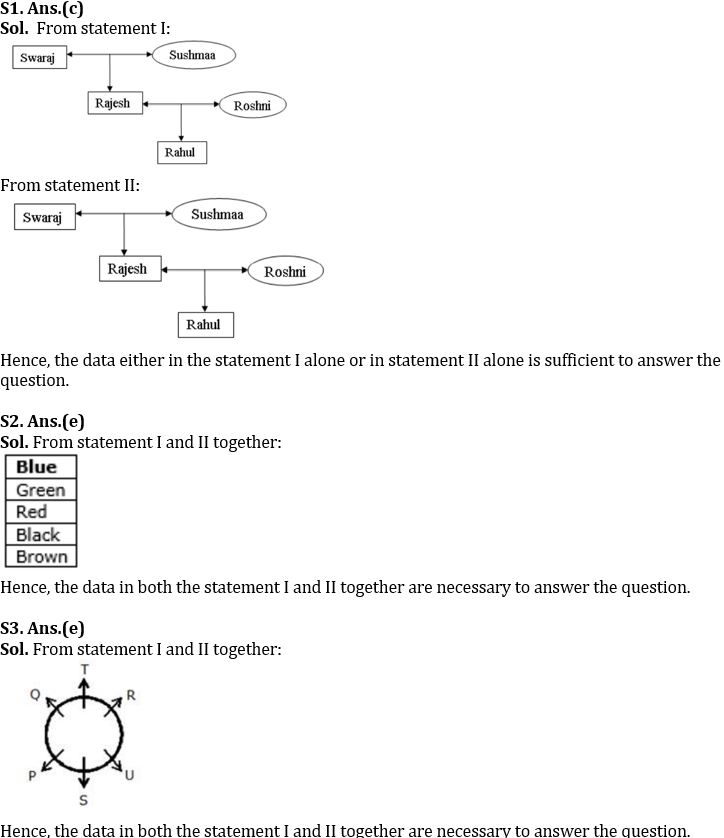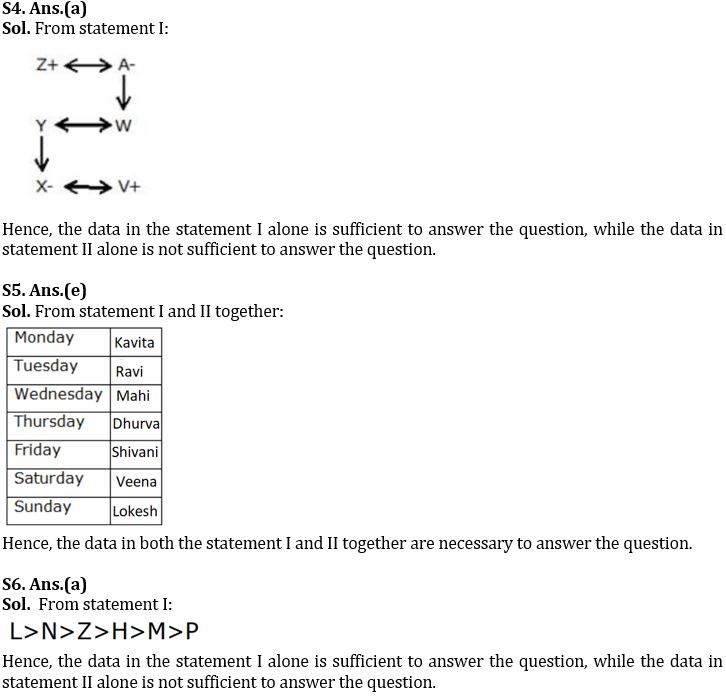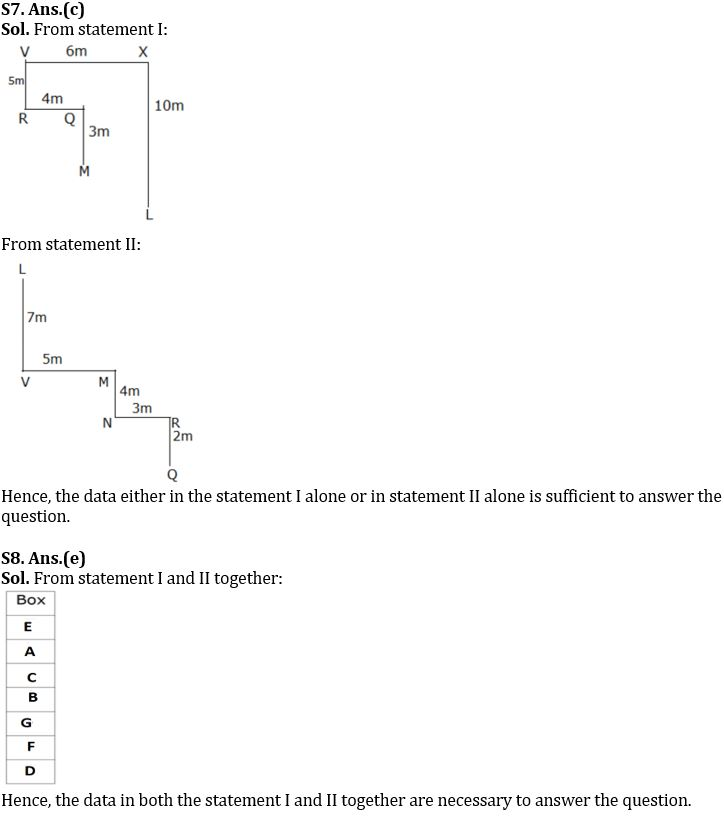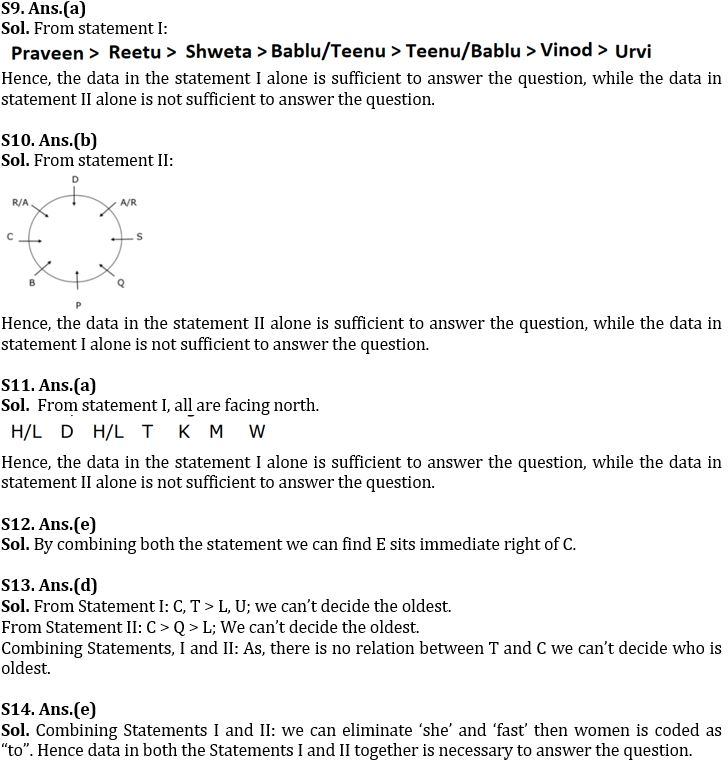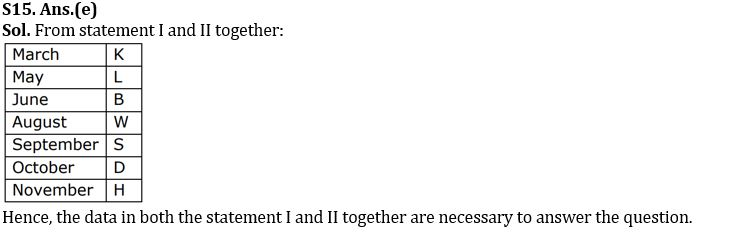#### Congratulations!Incorrect details? Fill the form again here

•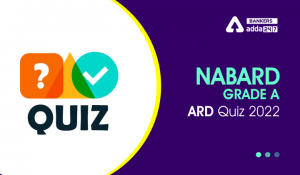Agriculture and Rural Development Quizze...
•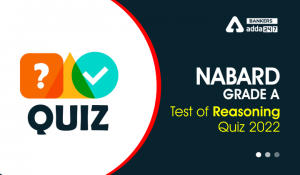Reasoning Ability Quiz For NABARD Grade ...
•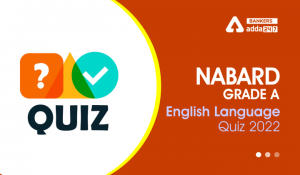English Quizzes For NABARD Grade A 2022-...
•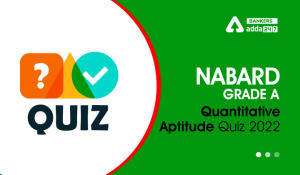Quantitative Aptitude Quiz For NABARD Gr...
•Reasoning Ability Quiz For NABARD Grade ...
•English Quizzes For NABARD Grade A 2022-...